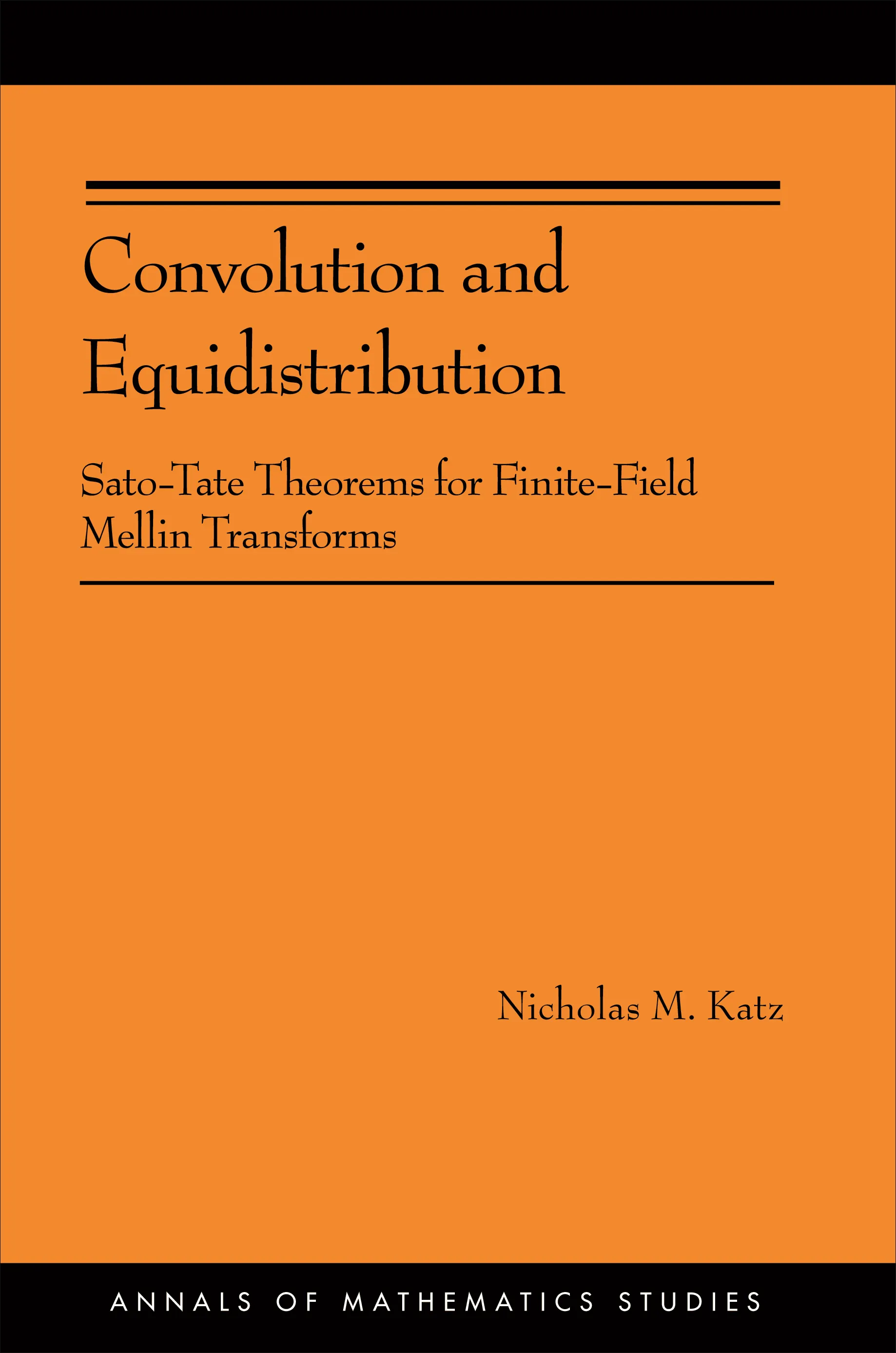#### Convolution and Equidistribution

Nicholas M. Katz

##### ebooks

Many of our ebooks are available for purchase from these online vendors:

Many of our ebooks are available through library electronic resources including these platforms:

# Convolution and Equidistribution: Sato-Tate Theorems for Finite-Field Mellin Transforms (AM-180)## Paperback

Price:
\$90.00 / £70.00
ISBN:
Published:
Jan 24, 2012
2012
Pages:
208
Size:
6 x 9.25 in.

Convolution and Equidistribution explores an important aspect of number theory — the theory of exponential sums over finite fields and their Mellin transforms — from a new, categorical point of view. The book presents fundamentally important results and a plethora of examples, opening up new directions in the subject.

The finite-field Mellin transform (of a function on the multiplicative group of a finite field) is defined by summing that function against variable multiplicative characters. The basic question considered in the book is how the values of the Mellin transform are distributed (in a probabilistic sense), in cases where the input function is suitably algebro-geometric. This question is answered by the book’s main theorem, using a mixture of geometric, categorical, and group-theoretic methods.

By providing a new framework for studying Mellin transforms over finite fields, this book opens up a new way for researchers to further explore the subject.